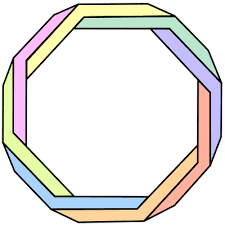Polygons
3 years ago
ktegman
Save
Edit
Host a game
Live GameLive
Homework
Solo Practice
Practice10 QuestionsShow answers
• Question 1
30 seconds
Q. A parallelogram with all sides of equal length is a ________.
triangle
trapezoid
rhombus
pentagon
• Question 2
30 seconds
Q. A quadrilateral with four sides of equal length and four right angles is ________.
square
rectangle
rhombus
octagon
• Question 3
30 seconds
Q. A _______  is a quadrilateral with two sides that are parallel but not of equal length.
pentagon
rhombus
square
trapezoid
• Question 4
30 seconds
Q. A _________ is a quadrilateral with two pairs of sides that are also of equal length.
triangle
circle
parallelogram
trapezoid
• Question 5
30 seconds
Q. I am a polygon.  I have more than one more vertex than a square has.  What am I?
triange
rectangle
pentagon
octagon
• Question 6
30 seconds
Q. A polygon with four sides is a __________.
triangle
decagon
octagon
• Question 7
30 seconds
Q. A _________ is a quadrilateral that has two pairs of parallel sides, two pairs of sides that are of equal length and four right angles.
rectangle
polygon
square
rhombus
• Question 8
30 seconds
Q. A right angle is ____ degrees.
180
100
85
90
• Question 9
30 seconds
Q. An octagon has _____ vertices.
1
4
6
8
• Question 10
30 seconds
Q. A hexagon has ___ angles.